IF YOU CAN SEE THIS go to /sfa/Nuprl/Shared/Xindentation_hack_doc.html

### Operator Definitions: Restrictions - continued from Operator Definition

Here are the restrictions we place on definitions.

First we consider some restrictions on a collection of definitions as a whole. Expansion must be well-founded, and no two definitions can be instantiated in such a way that the resulting left-hand sides are the same. This way there is no ambiguity about how to expand a term and repeated expansion must come to an end.

The left-hand side, the definiendum, must be "general" in the following ways.
A subterm in a position where no variables can become bound must be filled by a (first-order) variable, as in Def A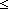B ==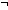B<A. The left-hand side of a definition could not be "1B" because the subterm "1" is not a variable.
A subterm in a position having binding variables must be an "instance" of a second-order variable whose subterms are the binding variables, and there are no duplicates among the binding variables or subterms of the second-order variable instance, as in Def Sym x,y:TE(x;y) ==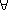a,b:TE(a;b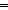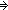E(b;a). It would not be possible to make a definition with left-hand side "Sym x,x:TE(x;x)" or "Sym x,y:TE(x;y;z)" or "Sym x,y:TE(x)".
Distinct subterm positions of the left-hand side must be filled by distinct variables.

Note that this condition on definienda together with the well-foundedness of definition expansion entail that an instance of the left-hand side of a definition cannot occur on the right-hand side of the definition, since that would cause an expansion cycle. Recursive Functions and Recursive Types are defined by other means.

On the left-hand side an op-parm place (see Terms) may be filled either by an op-parm variable (see Operator Definition) as in Def \$abc.\$n == <"\$abc",\$n>, or by a constant. That is, one is not permitted to use a complex op-parm expression on the left if it contains a variable, so for example, "HigherConsequence{k'}(P)" cannot be the left side of a definition since the level expression is a complex level-expression containing a variable.
Note that one can use op-parm constants to distinguish methods of expansion. For example, the following can coexist:

Def sfa_doc_oparm_sample{1:n}(A) ==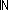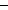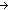A

Def sfa_doc_oparm_sample{2:n}(A) == A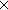The right-hand side (definiens) of a definition is constrained by the left-hand side (definiendum). All the first order variables occurring free, all the second-order variables occurring, and all the op-parm variables occurring in the right-hand side must occur in the left-hand side. So, all the (free) variables in a term resulting from expanding definitions must come from the original term, and cannot have been introduced by the definitions.

Thus, one never has to examine the contents of definitions in order to know what the free variables or the level variables of a term are, which is key since as a result one can usually recognize instances of inference rules independently of operator definitions.

(May 2001 - sfa )